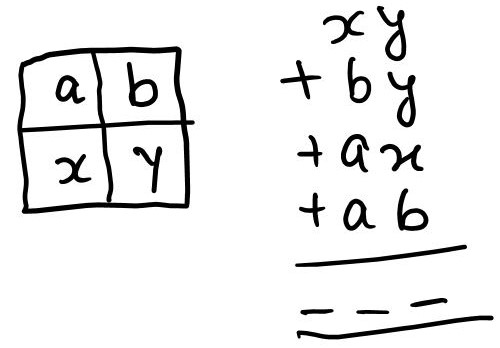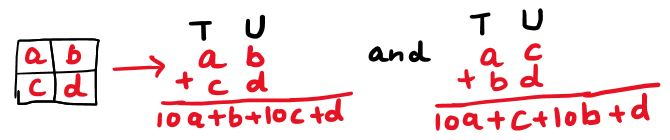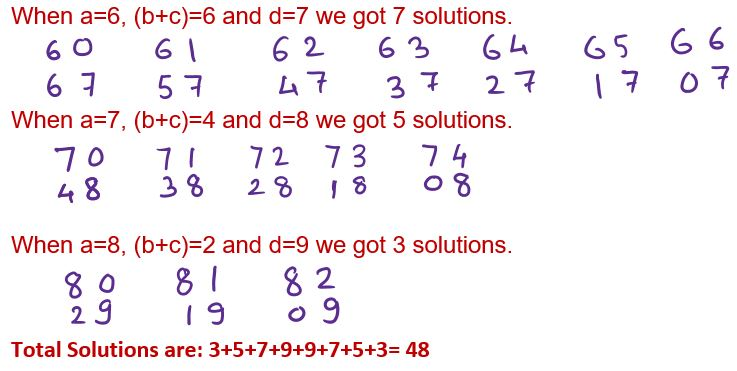#### You may also like### Double Digit### Repeaters

Choose any 3 digits and make a 6 digit number by repeating the 3 digits in the same order (e.g. 594594). Explain why whatever digits you choose the number will always be divisible by 7, 11 and 13.### Big Powers

Three people chose this as a favourite problem. It is the sort of problem that needs thinking time - but once the connection is made it gives access to many similar ideas.

##### Age 11 to 14Challenge Level

Niveditha, Avyukt and Rudransh from Ganit Kreeda, Vicharvatika in India searched for patterns when adding the numbers. This is Avyukt's observation:

While experimenting with different examples, [I] noticed that by increasing bottom rightmost digit by 1 the sum is increased by 2.

 5 3 5 5

53 + 55 = 108, 55 + 35 = 90
108 + 90 = 198

 5 3 5 6

53 + 56 = 109, 55 + 36 = 91
109 + 91 = 200

Rudransh explained why this happens:

Digit 6 is at unit’s place in both vertical and horizontal addition. So, it changes sum by 2.

Niveditha thought about whether the total was even or odd. Can you see how Niveditha's work also explains what Avyukt noticed?Niveditha noticed that units place digits are $y,y,x$ and $b.$ Whatever is $y,$ as $y$ is used twice, $2y$ is always even. So the sum is even or odd if $(x+b)$ is even or odd respectively.

Sowjanya, Avyukt Aggrawal, Adhrit, Achyuth, Ridhit, Niveditha, Pranit, Taran, Avyukt Aswin, Rudransh, Abhiram, Nishika, Vidyut, Vishnu, Ruhaan and Subhangee from Ganit Kreeda, Vicharvatika, Sofia from Colyton Grammar in England, Joshua and James from RMH Hospital Schoolroom in the UK and Teppi Ta from BVIS Ho Chi Minh City in Vietnam found numbers which make a total of 200.

Pranit found these examples:

Pranit tried to find out pairs of 100, such that units place of one number is same as tens place of second number such as 81+19; 63+37

 8 1 1 9

 6 3 3 7

Sofia found the same examples, and more, but she thought about them in a different way. Can you see why they give the same answers Pranit found?

The first number has to be 1 smaller than the fourth, then take the fourth number away from 10 and the number that you are left with should fill the second and third place.

Examples:

2    7
7    3

3    6
6    4

0    9
9    1

4    5
5    5

5    4
4    6

6    3
3    7

Joshua and James explored examples that have the same number in the top left box.

James found this solution-

4  3
7  5

and then worked out that the digits were worth different values depending on their position in the grid. He found that he could alter his solution by changing the numbers as follows:

Top left = +/- 20 (2 tens)
Top right = +/- 11 (1 ten and 1 one)
Bottom left = +/- 11 (1 ten and 1 one)
Bottom right = +/- 2 (two ones)

Because the top right and bottom left numbers are worth the same amount, they can be swapped, or as Josh said "balanced"- increasing one number and decreasing the other by the same amount, without changing the total.

He used this to show that there would be 9 solutions with 4 in the top left and 5 in the bottom right, with the other two numbers adding up to 10.

Teppi Ta and the students from Ganit Kreeda used algebra to find all of the possible combinations! This is the work done by the students at Ganit Kreeda:

Rudransh’s reason was explained using algebra.

$a$   $b$
$c$   $d$

The numbers to add are $10a+b, 10c+d, 10a+c$ and $10b+d.$

$20a + 11(b + c) + 2d = 200$

It is clear that :
$a$ cannot be $9,$ as $180 + 20$ is $200.$ [If $b+c=0$ to give $11\times0$ then $d$ would have to be $10$] and $d$ cannot be $10$
[If $b+c=1$ or 2 then] $180 + 11 + 2d$ or $180 + 22 + 2d$ will not add to 200.

$a$ can take values from $1$ to $8.$

when $a = 8,$ $(b + c)$ can only be $2$ and $d = 9$

when $a = 7,$ $(b + c)$ can only be $4$ and $d = 8$

and so on...Teppi Ta found some more patterns:

1st, all of the numbers should add up to 19 (all 4 numbers)
2nd, the first number (the number in the upper left of the box) will always be less than one with the number in the lower right of the box)
3rd, the numbers in the other boxes (that have not been mentioned yet) can change places with each other)

The students from Ganit Kreeda also investigated the final question, which numbers between 0 and 396 is it possible to make?

It is not possible to make 1,3,5,7 and 9…single digit odd numbers.

All other numbers are possible.

0,2,4,6,8,10,12,14,16,18 are possible by assigning $d$ = 0,1,2…..9.

11,13,15,17,19,21…29 are possible by assigning ($b$+$c$)=1 and $d$=0,1,2,3….9

20,22,..,38 are possible by assigning $a$=1 and $d$=0,1,2,3….9

31,33,..,49 are possible by assigning $a$=1, ($b$+$c$)=1 and $d$=0,1,2,3….9

And so on.